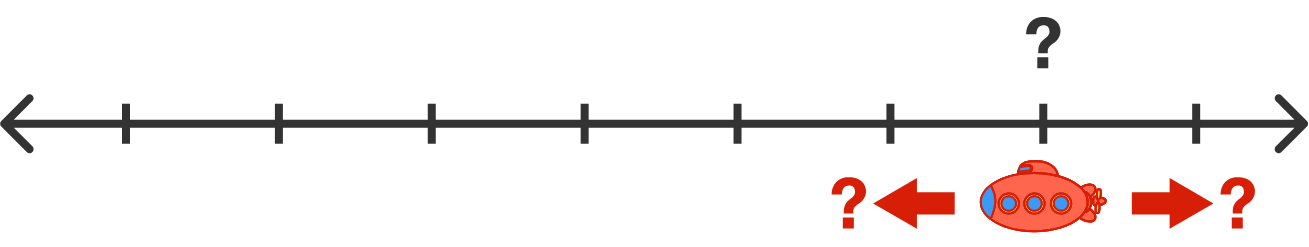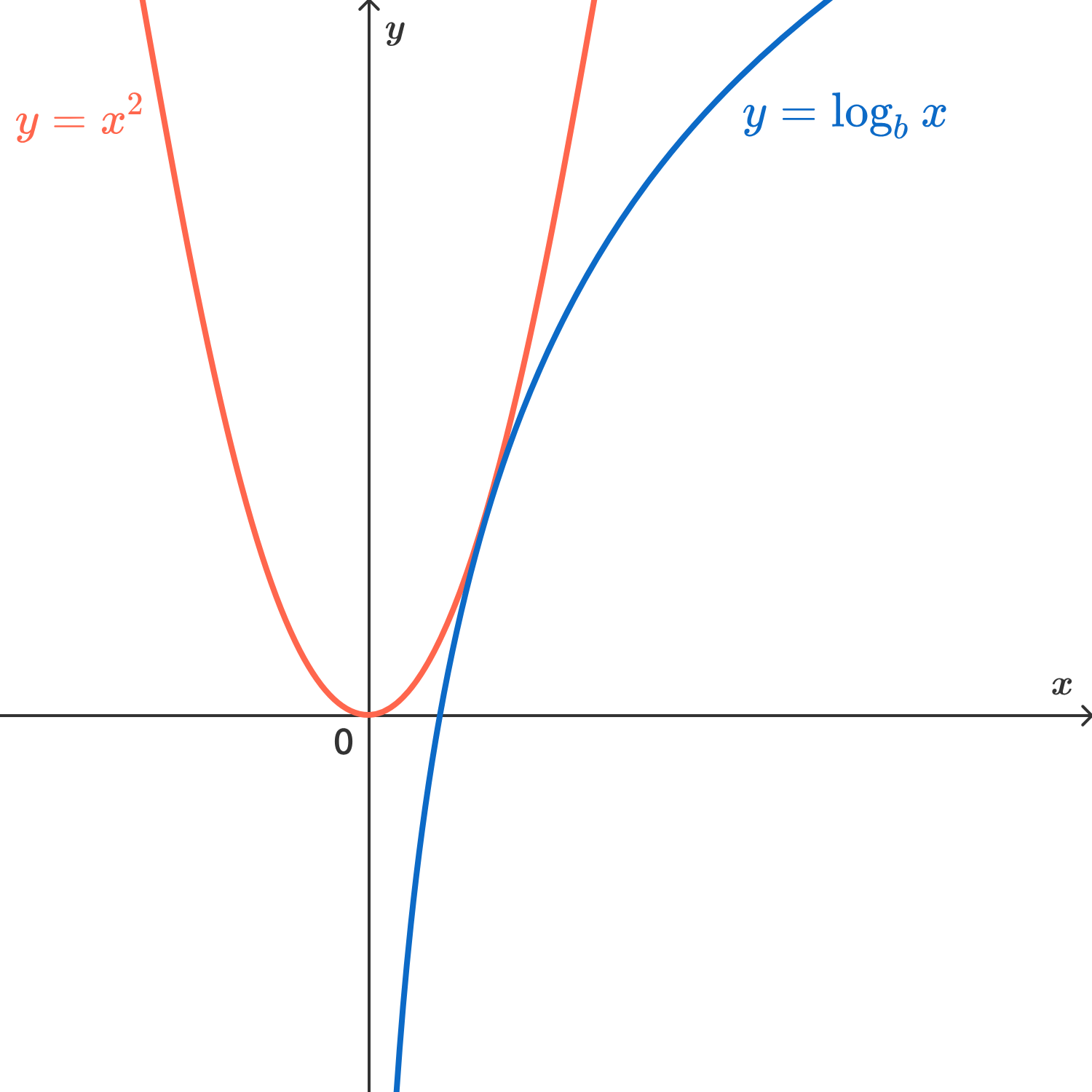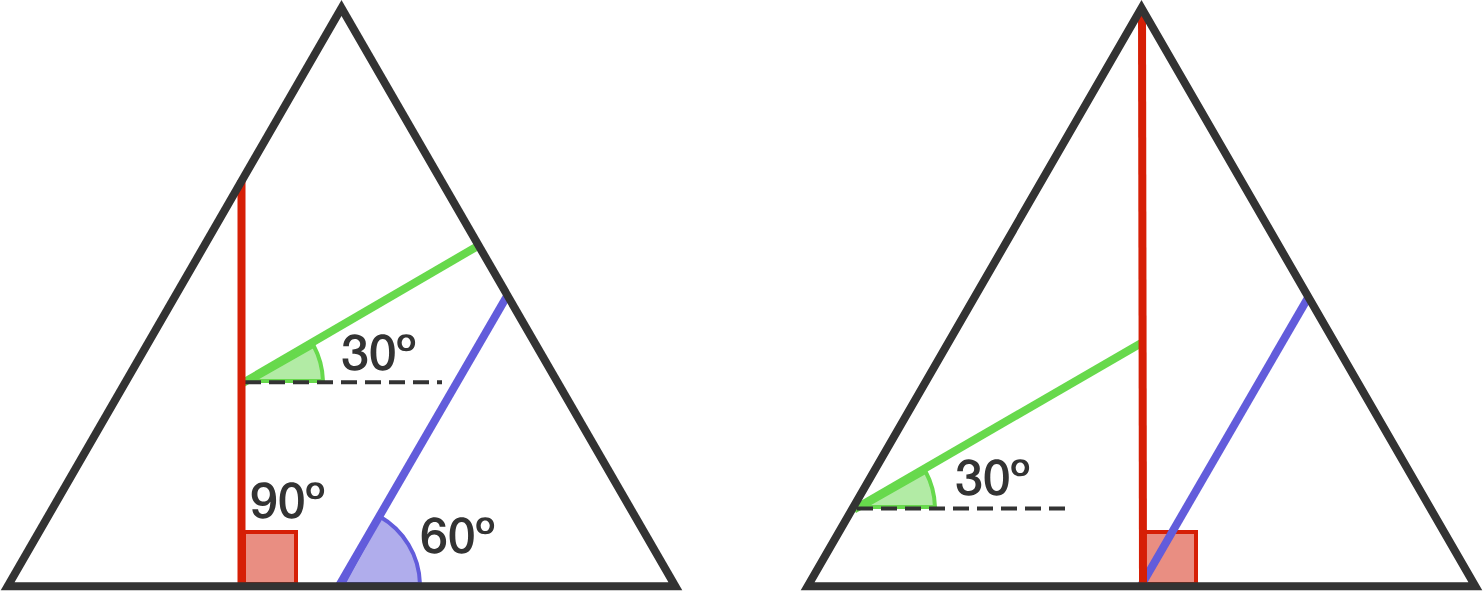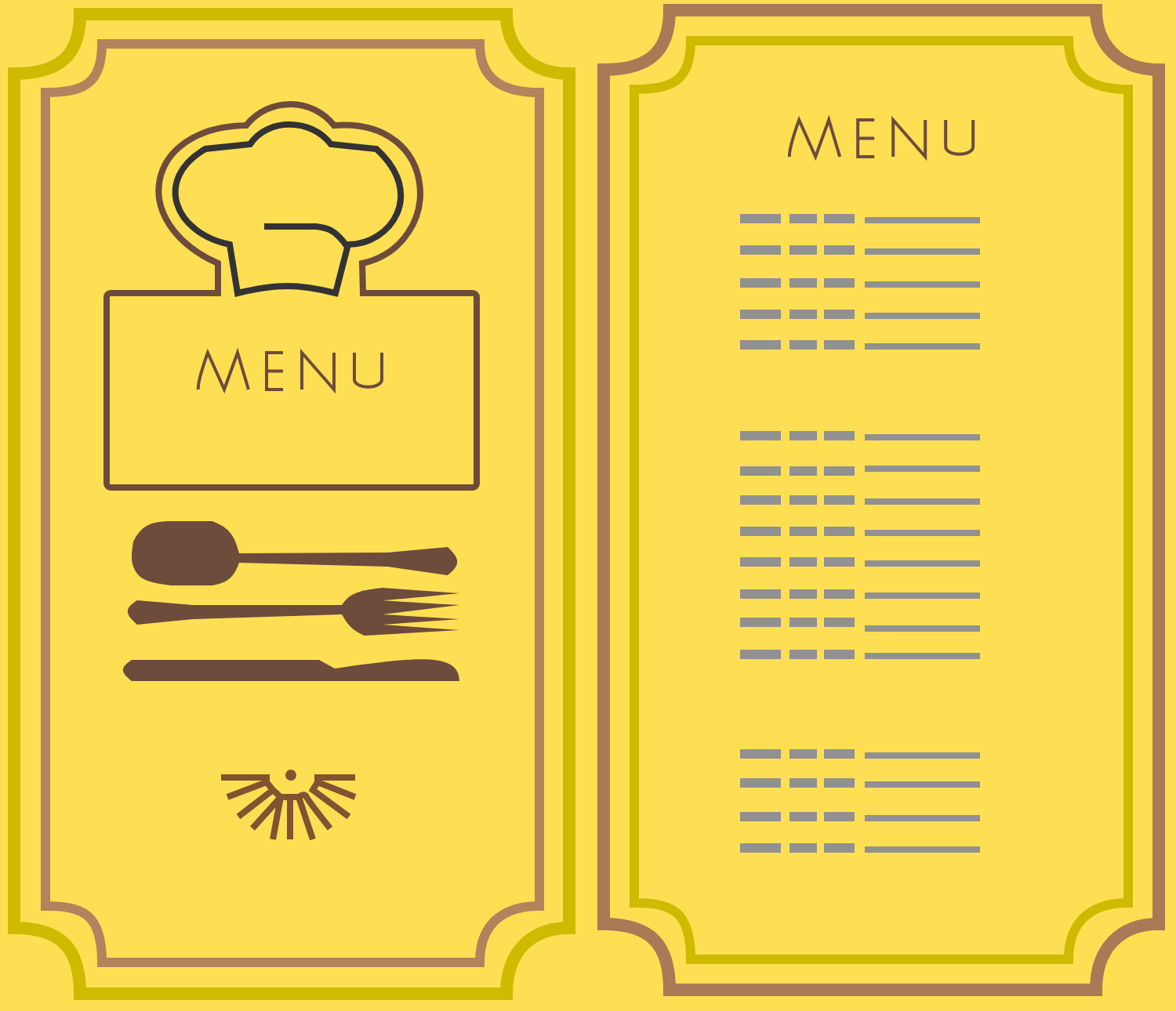# Problems of the Week

Contribute a problem

There is an enemy submarine located at an unknown integer somewhere on the number line. The submarine is moving at a constant integer distance per minute (also unknown, and can be positive or negative). Every minute, you can fire a missile at an integer on the number line in an attempt to destroy the submarine.

Is there a strategy to strike the submarine in a finite amount of time?

Assume that you move first and that futuristic medical science allows you to continue firing missiles at the number line for as long you need to.$b$ is a real number greater than 1 such that the equation $x^2 = \log_b x$ has exactly one real solution.

Submit $\big\lfloor 10000 \{ b \} \big\rfloor,$ where $\{ \cdot \}$ and $\lfloor \cdot \rfloor$ denote the fractional part function and floor function, respectively.The curves only touch once.

A spherical star has a (volumetric mass) density of $\rho(r)=3-r,$ where $r$ is the distance from the star's center, for $0\leq r \leq 3.$

At what distance from the center is the star's gravitational field the strongest?

Mr. Lefty plans to cut an equilateral-triangle-shaped pizza into four parts of congruent areas. He does this with three cuts made at angles $30^\circ,$ $60^\circ,$ and $90^\circ,$ each measured counterclockwise from the horizontal. The diagram below shows two examples of how the pizza may be cut.The dashed lines are there to show how the cuts not touching the horizontal segment are made.

In total, how many ways are there to cut the pizza (including the two ways shown above)?A restaurant has $N \ge 4$ dishes on its menu that are each distinct in quality, rated from $1$ (the worst) to $N$ (the best). You do not know the quality rating of each dish, although you can judge a better dish after eating two different dishes.

You plan to visit the restaurant 4 times this week, and your goal is to maximize the average quality rating of the 4 dishes that you order. For the first $n$ days, you order a different random dish each day. Then, for the next $4-n$ days, you order the best dish out of the dishes that you have already tried.

What value of $n$ maximizes the average expected quality rating of the dishes that you order?

×